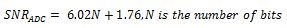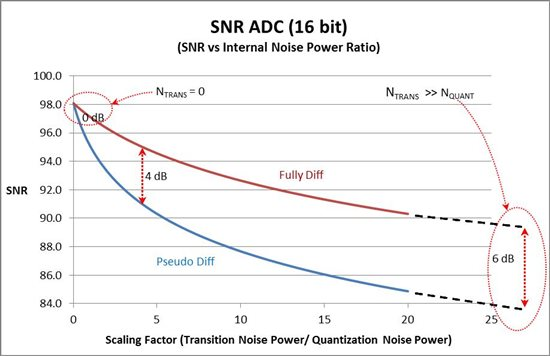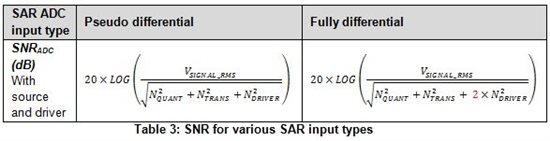# Performance comparison between SAR ADC input types – Part 1

This technical article was updated on July 23, 2020.

My previous blog Input considerations for SAR ADC's, introduced various input types for Successive Approximation Register (SAR) analog-to-digital converters (ADCs): single-ended, pseudo-differential, fully differential input and true differential input.

Some of the key specifications considered when selecting a SAR ADC include resolution, channel count, sampling rate, power supply range, power consumption, digital interface and clock speeds. But what about noise and AC parameters such as Signal-to-Noise Ratio (SNR) and Total Harmonic Distortion (THD)? These parameters can affect the overall system performance, and hence, SAR input-type selection.

System Noise and GND Difference Impacts

Single-ended input: These SAR ADCs require only one trace/cable and a single input driver, if any, to connect to the source. It is important to note that these ADC's measure input signal with respect to the SAR ADC's own ground. While this is the simplest configuration, voltage potential differences between the signal ground and SAR ADC's ground will affect the conversion result and accuracy. Also, noise coupled from supply and ground into the internal sampling capacitor will affect conversion accuracy as the common mode rejection (CMRR) is poor to non-existent.

Differential inputs (Pseudo, Fully Differential and True Differential): While this requires an additional trace/cable and two input drivers, the benefits include:

• With proper layout techniques, coupling from supply noise, ground bouncing, clocks etc. on the two inputs will be similar. Better CMRR significantly reduces any common-mode noise that is correlated between the two inputs.
• For pseudo-differential input in particular, the negative input can connect to the signal ground or offset, thereby eliminating conversion results due to voltage differences between signal ground and SAR ground.

Examples: Refer to ‘operating input range’ for AINM in the specification table for devices such as ADS7042, ADS8860 and ADS8319.

SNR Impact

An ADC’s SNR compares the signal power component to the noise power below one-half the sampling frequency, not including harmonics and DC. SNR impact is computed using the following equations:

The total system noise at the input of the ADC is composed of two components: external noise coupled from the signal source and input driver and internal noise at the ADC input. Since single-ended architectures (having one CDAC structure) are rare, I’ll compare performance differences between pseudo-differential (using one CDAC structure on each input) and fully-differential inputs.

For a given architecture, the input signal range on a fully-differential SAR (–REF to REF) is double that of pseudo-differential SARs (0 – REF). The quantization noise introduced for an AC signal due to rounding of the signal to the closest code also doubles for fully differential input*.
*(BSTJ 27:3. July 1948: Spectra of Quantized Signals. (Bennett, W. R.) (July 1948)

Internal Noise: To analyze the SNR of an ADC, we first assume an ideal AC source and driver with negligible noise. The impact of internal noise can be analyzed using quantization noise (NQUANT) and transition noise (NTRANS) fundamental to ADC.

For an ideal ADC with only quantization error (i.e. NTRANS = 0), SNRADC will be same for both input types as shown in Table 2. It is given by the equation:Transition noise is introduced by the active circuitry such as comparators and by kT/C noise from resistors and capacitors. As NTRANS dominates, improvement up to 6dB can be seen in the SNRADC for fully-differential ADCs as shown in Table 2. This is due to the dynamic range doubling while NTRANS remains same.

Below, Figure 1 better illustrates this situation, showing SNRADC for a 16-bit ADC. The SNRADC for both input types is same for ideal ADC. As NTRANS starts to scale, the difference between the two SNR’s widens. With NTRANS >> NQUANT, 6dB change can be seen. In practice, this difference in SNRADC is anywhere between 0 dB to 6 dB depending on how design optimizes the ratio of NTRANS to NQUANT.

Figure 1: SNR comparison with internal noise